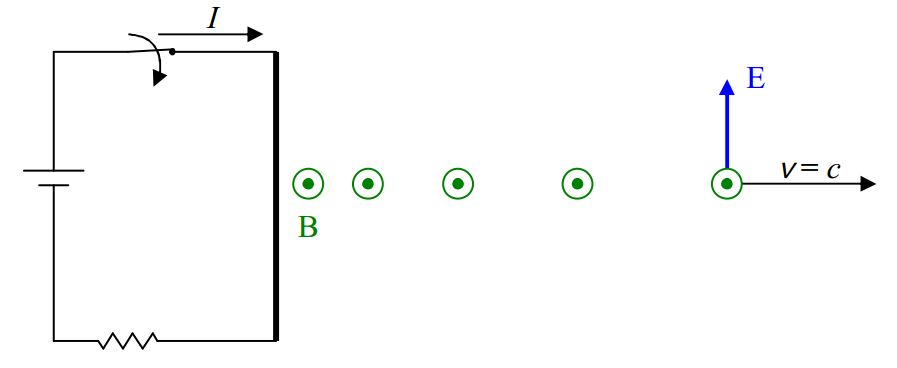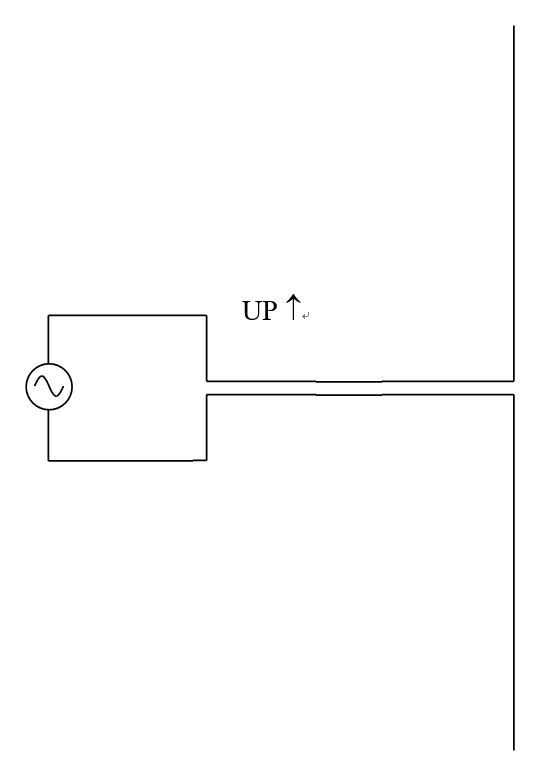$$\require{cancel}$$

# B21: The Nature of Electromagnetic Waves

When we left off talking about the following circuit:we had recently closed the switch and the wire was creating a magnetic field which was expanding outward. The boundary between that part of the universe in which the magnetic field is already established and that part of the universe in which the magnetic field has not yet been established is moving outward at the speed of light, $$c=3\times 10^8 m/s$$. Between that boundary and the wire we have a region in which there exists a steady unmoving magnetic field. Note that it was the act of creating the current that caused the magnetic field “edge” that is moving at the speed of light. In changing from a no-current situation to one in which there was current in the wire, charged particles in the wire went from no net velocity in the along-the-wire direction to a net velocity along the wire, meaning, that the charged particles were accelerated. In other words, accelerated charged particles cause light. We can also cause light by means of the angular acceleration of particles having a magnetic dipole moment, but, the short answer to the question about what causes light, is, accelerated charged particles.

Here’s a simple circuit that one might use to intentionally cause light:The vertical arrangement of wires on the right is referred to as a dipole antenna. As the $$AC$$ power source alternately causes charge to surge upward in both parts of the antenna, and then downward, the dipole antenna creates electric and magnetic fields that oscillate sinusoidally in both time and space. The fields propagate through space away from the antenna at the speed of light.

The charged particles oscillating up and down in the antenna causes waves of electric and magnetic fields known as light. The frequency of the waves is the same as the frequency of oscillations of the particles which is determined by the frequency of the power source. The speed of the waves is the speed of light $$c=3\times 10^8 m/s$$, because the waves are light. For any kind of wave, the frequency, wavelength, and wave speed are related by:

$v=\lambda f$

which, in the case of light reads:

$c=\lambda f$

Here’s a quick pictorial review of some properties of waves. In the case of light, we have electric and magnetic fields oscillating in synchronization with each other. It is customary to characterize the waves in terms of the electric field. I’ll do that here, but, one should keep in mind that the magnetic field oscillates and moves in the same manner that the electric field does, but, at right angles to the electric field.The intensity of a wave is proportional to the square of its amplitude, so, in the case of light:

$I\propto (E_{MAX})^2$

The frequency of light is determined by the frequency of oscillations of the charged particles constituting the source of the light. How we categorize light depends on the frequency of the light. In order of increasing frequency, we refer to light as: radio waves, microwaves, infrared radiation, visible light, ultraviolet light, X rays, and gamma rays. They are all the same thing— electric and magnetic fields that are oscillating in time and space. I am using the word light in a generic sense. It refers to waves of any one of these various frequencies of oscillations of electric and magnetic fields. In this context, if I want to talk about light whose frequency falls in the range to which our eyes are sensitive, I refer to it as visible light. Another name for light is electromagnetic radiation. The entire set of the different frequencies of light is referred to as the electromagnetic spectrum. The following table indicates the way in which humans categorize the various frequencies of light in the electromagnetic spectrum. While I do give definite values, boundaries separating one frequency from the next are not well defined and hence, should be treated as approximate values.

 Kind of Light Frequency Wavelength Radio Waves $$< 300 \, MHz$$ $$> 1\space m$$ Microwaves $$300-750 000 \, MHz$$ $$.4 \, mm - 1 \, m$$ Infrared $$750 \, GHz -430 \, GHz$$ $$700 \, nm - .4 \space mm$$ Visible $$430-750 \, THz$$ $$400-700 \space nm$$ Ultraviolet $$750-6000 \space THz$$ $$5-400 \, nm$$ X rays $$6000-50 000 000 \, THz$$ $$.006-5 \, nm$$ Gamma Rays $$> 50 000 000 \space THz$$ $$< .006\space nm$$

Note that the visible regime is but a tiny slice of the overall electromagnetic spectrum. Within it, red light is the long-wavelength, low-frequency visible light, and, blue/violet light is the short-wavelength high-frequency visible light. AM radio stations broadcast in the $$kHz$$ range and FM stations broadcast in the $$MHz$$ range. For instance, setting your AM dial to $$100$$ makes your radio sensitive to radio waves of frequency $$100\space kHz$$ and wavelength $$3000\space m$$. Setting your FM dial to $$100$$ makes your radio sensitive to radio waves of frequency $$100\space MHz$$ and wavelength $$3\space m$$.

We call the superposition of the changing electric and magnetic field vectors, with other changing electric and magnetic field vectors, interference. Many of the phenomena involving light are understood in terms of interference.

When light interacts with matter, its electric field exerts forces on the charged particles that make up matter. The direction of the force exerted on a charged particle is the same direction as the electric field if the particle is positive, and in the opposite direction if it is negative. The magnetic field exerts a torque on the magnetic-dipole-possessing particles that make up matter. Because so many of the observable effects associated with the interaction of visible light have to do with the force exerted on charged particles by the electric field, it has become customary to talk about the interaction of light with matter in terms of the interaction of the electric field with matter. I will follow that custom. Please keep in mind that the magnetic field, always at right angles to the electric field in light, is also present. As a result of the force exerted on the charged particles by the electric field of which the light consists, the charged particles accelerate, and, as a result, produce their own electric and magnetic fields. Because there is no time delay between the exertion of the force and the resulting acceleration, the newly produced electric and magnetic field vectors superpose with the very electric and magnetic field vectors causing the acceleration. Because the mass of an electron is approximately $$1/2000$$ of the mass of a proton, the acceleration experienced by an electron is $$2000$$ times greater than that experienced by a proton subject to the same force. Hence, the interaction of light with matter, can often be explained in terms of the interaction of light with the electrons in matter.

How the electrons in matter interact with light, is largely determined by the degree to which the electrons are bound in the matter. As a rather bizarre example of how a large number of complicated interactions can combine to form a simple total effect, the mix of attractive and repulsive $$1/r^2$$ Coulomb forces exerted on the electron in a solid material by the protons and electrons on all sides of it, results in a net force on the electron that is well modeled by the force that would be exerted on a particle “tied” to its equilibrium position by a spring. Hence the electron acts like a “mass on a spring.” As such, it can undergo simple harmonic motion like a mass on the end of a spring. The way light interacts with the electrons can thus be said to depend on the frequency of the light and the force constant of the effective spring. If we limit our discussion to visible light, the degree to which the electrons are bound (the spring constant) determines how the light interacts with the matter. In the case of what we would consider opaque light-absorbing matter such as flat black paint, the electron accelerations result in destructive interference of the incoming light with the light produced by the electrons. Light doesn’t go through, nor is much reflected off the material. In the case of shiny metal surfaces, the electrons that the light interacts with are virtually free. The light emitted by these electrons as a result of the acceleration caused by the light, interferes constructively with the light in a very specific backward direction and destructively in forward directions. Hence, the light does not get through the metal, but, it is reflected in a mirror-like manner referred to as specular reflection. In the case of a transparent medium such as glass, the light given off by the electrons interferes with the incoming light in such a manner as to cause constructive interference in specific forward and backward directions. But, the constructive interference in the forward direction is such that the pattern of electric and magnetic waves formed by all the interference taken as a whole, moves more slowly through the glass than light moves through vacuum. We say that the speed of light in a transparent medium is less than the speed of light in vacuum. The ratio of the speed of light in vacuum to the speed of light in a transparent medium is called the index of refraction, $$n$$, of that transparent medium.

$n=\frac{c}{v}$

where:

$$n$$ is the index of refraction of a transparent medium,

$$c=3.00 \times 10^8 \, m/s$$ is the speed of light in vacuum, and,

$$v$$ is the speed of light in the transparent medium.

Because the speed of light never exceeds the-speed-of-light-in-vacuum, the index of refraction is always greater than or equal to 1 (equal when the medium is, or behaves as, vacuum). Some values for the index of refraction of light for a few transparent media are:

 Medium Index of Refraction Vacuum 1 Air 1.00 Water 1.33 Glass (Depends on the kind of glass. Here is one typical value). 1.5

The kind of interaction of light with matter with which we are most familiar is called diffuse reflection. It is the light that is diffusely reflecting off a person that enters your eyes when you are looking at that person. The electron motion produces light that interferes destructively with the incoming light in the forward direction (the direction in which the incoming light is traveling), so, essentially none gets through but, for a particular frequency range, for all backward directions, very little destructive interference occurs. When the object is illuminated by a mix of all visible frequencies (white light), the frequency of the reflected light depends on the force constant of the effective spring that is binding the electrons to the material of which they are a part. The frequency reflected (in all backward directions) corresponds to what we call the color of the object.